Space and Time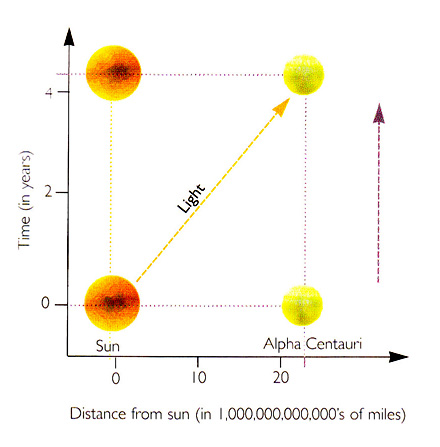Fig. 2.9  Space-time diagram showing a light signal (diagonal line) going from the sun to Alpha Centauri. The paths of the sun and Alpha Centauri through space-time are straight lines.

Maxwell's equations predicted that the speed of light should be the same whatever the speed of the source, and this has been confirmed by accurate measurements. It follows from this that if a pulse of light is emitted at a particular time at a particular point in space, then as time goes on it will spread out as a sphere of light whose size and position are independent of the speed of the source. After one millionth of a second the light will have spread out to form a sphere with a radius of 300 meters; after two millionths of a second, the radius will be 600 meters; and so on. It will be like the ripples that spread out on the surface of a pond when a stone is thrown in. The ripples spread out as a circle that gets bigger as time goes on. If one stacks snapshots of the ripples at different times one above the other, the expanding circle of ripples will mark out a cone whose tip is at the place and time at which the stone hit the water (Figure 2:10)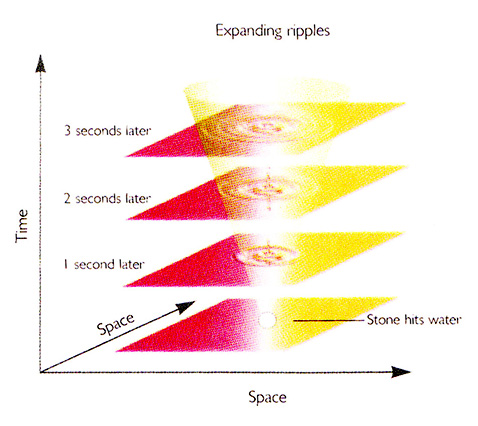Fig. 2.10  A space-time diagram showing ripples spreading on the surface of a pond. The expanding circle of ripples makes a cone in space-time of two space directions and one time direction.

Similarly, the light spreading out from an event forms a (three- dimensional) cone in (the four-dimensional) space-time. This cone is called the future light cone of the event. In the same way we can draw another cone, called the past light cone, which is the set of events from which a pulse of light is able to reach the given event (Figure 2:11)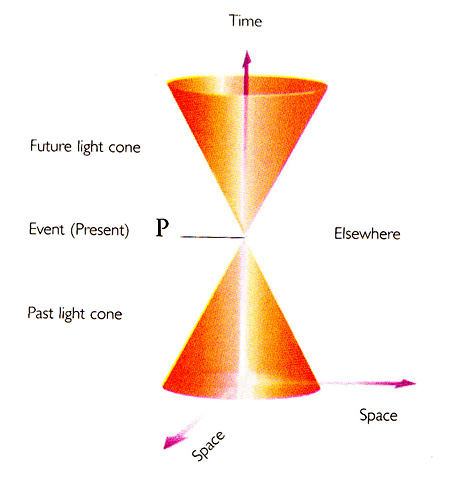Fig. 2.11  The path of a pulse of light from an event P forms a cone in space-time called "the future light cone of P." Similarly, "the past light cone of P" is the path of rays of light that will pass through the event P. The two light cones divide space-time into the future, the past and the elsewhere of P.

Given an event P, one can divide the other events in the universe into three classes. Those events that can be reached from the event P by a particle or wave traveling at or below the speed of light are said to be in the future of P. They will lie within or on the expanding sphere of light emitted from the event P. Thus they will lie within or on the future light cone of P in the space-time diagram. Only events in the future of P can be affected by what happens at P because nothing can travel faster than light.

Similarly, the past of P can be defined as the set of all events from which it is possible to reach the event P traveling at or below the speed of light. It is thus the set of events that can affect what happens at P. The events that do not lie in the future or past of P are said to lie in the elsewhere of P. What happens at such events can neither affect nor be affected by what happens at P. For example, if the sun were to cease to shine at this very moment, it would not affect things on earth at the present time because they would be in the elsewhere of the event when the sun went out (Figure 2:12)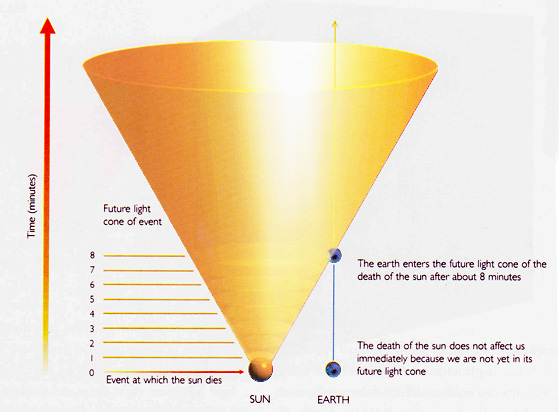Fig. 2.12   Space-time diagram showing how long we would have to wait to know that the sun has died.

We would know about it only after eight minutes, the time it takes light to reach us from the sun. Only then would events on earth lie in the future light cone of the event at which the sun went out. Similarly, we do not know what is happening at the moment farther away in the universe: the light that we see from distant galaxies left them millions of years ago, and in the case of the most distant object that we have seen, the light left some eight thousand million years ago. Thus, when we look at the universe, we are seeing it as it was in the past.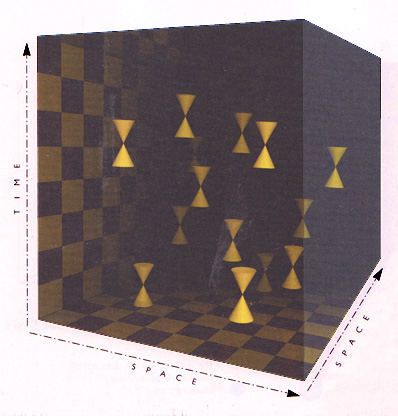Fig. 2.13  When the effects of gravity are neglected, the light cones of all events all point in the same direction.